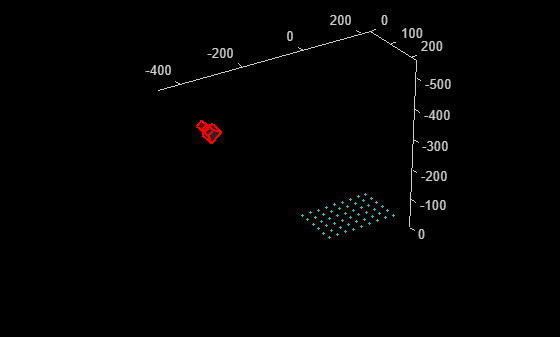# extrinsics

Compute location of calibrated camera

## Syntax

``````[rotationMatrix,translationVector] = extrinsics(imagePoints,worldPoints,cameraParams)``````

## Description

example

``````[rotationMatrix,translationVector] = extrinsics(imagePoints,worldPoints,cameraParams)``` returns the 3-D rotation matrix and the 3-D translation vector to allow you to transform points from the world coordinate to the camera coordinate system.```

## Examples

collapse all

Create a set of calibration images.

``` images = imageDatastore(fullfile(toolboxdir('vision'),'visiondata', ... 'calibration', 'slr'));```

Detect the checkerboard corners in the images.

`[imagePoints,boardSize] = detectCheckerboardPoints(images.Files);`

Generate the world coordinates of the checkerboard corners in the pattern-centric coordinate system, with the upper-left corner at (0,0). The square size is in millimeters.

```squareSize = 29; worldPoints = generateCheckerboardPoints(boardSize, squareSize);```

Calibrate the camera.

```I = readimage(images,1); imageSize = [size(I,1), size(I,2)]; cameraParams = estimateCameraParameters(imagePoints,worldPoints, ... 'ImageSize',imageSize);```

```imOrig = imread(fullfile(matlabroot,'toolbox','vision','visiondata', ... 'calibration','slr','image9.jpg')); figure imshow(imOrig); title('Input Image');```Undistort image.

`[im,newOrigin] = undistortImage(imOrig,cameraParams,'OutputView','full');`

Find reference object in new image.

`[imagePoints,boardSize] = detectCheckerboardPoints(im);`

Compensate for image coordinate system shift.

```imagePoints = [imagePoints(:,1) + newOrigin(1), ... imagePoints(:,2) + newOrigin(2)];```

Compute new extrinsics.

```[rotationMatrix, translationVector] = extrinsics(... imagePoints,worldPoints,cameraParams);```

Compute camera pose.

```[orientation, location] = extrinsicsToCameraPose(rotationMatrix, ... translationVector); figure plotCamera('Location',location,'Orientation',orientation,'Size',20); hold on pcshow([worldPoints,zeros(size(worldPoints,1),1)], ... 'VerticalAxisDir','down','MarkerSize',40);```## Input Arguments

collapse all

Image coordinates of points, specified as an M-by-2 array. The array contains M number of [x, y] coordinates. The `imagePoints` and `worldPoints` inputs must both be `double` or both be `single`.

Data Types: `single` | `double`

World coordinates corresponding to image coordinates, specified as an M-by-2 matrix. The `imagePoints` and `worldPoints` inputs must both be `double` or both be `single`. The function assumes that the points are coplanar with z= 0 and the number of points, M, must be at least 4.

Data Types: `single` | `double`

Object for storing camera parameters, specified as a `cameraParameters`, `cameraIntrinsics`, or `fisheyeIntrinsics` object. These objects are returned by the `estimateCameraParameters` function, the `estimateFisheyeParameters` function, or the Camera Calibrator app. The object contains the intrinsic, extrinsic, and lens distortion parameters of a camera.

## Output Arguments

collapse all

3-D rotation, returned as a 3-by-3 matrix. The rotation matrix together with the translation vector allows you to transform points from the world coordinate to the camera coordinate system.If you set the `imagePoints` and `worldPoints` inputs to class `double`, then the function returns the `rotationMatrix` and `translationVector` as `double`. Otherwise, they are `single`.

3-D translation, returned as a 1-by-3 vector.If you set the `imagePoints` and `worldPoints` inputs to class `double`, then the function returns the `rotationMatrix` and `translationVector` as `double`. Otherwise, they are `single`.

## Algorithms

The `extrinsics` function uses two different algorithms to compute the extrinsics depending on whether `worldPoints` are specified as an M-by-2 matrix. Use an M-by-2 matrix for coplanar points where z= 0.

The `extrinsics` function computes the rotation matrix and translation vector for a single image in closed form. During calibration, the extrinsics are estimated numerically to minimize the reprojection errors for all calibration images. Therefore, using the `extrinsics` function on one of the calibration images returns rotation matrix and translation vector slightly different from the ones obtained during calibration.